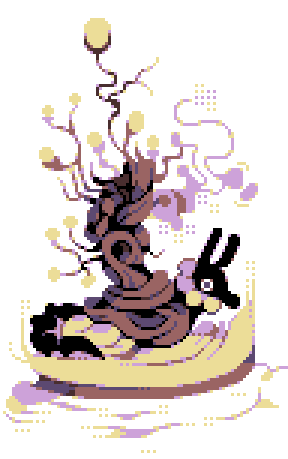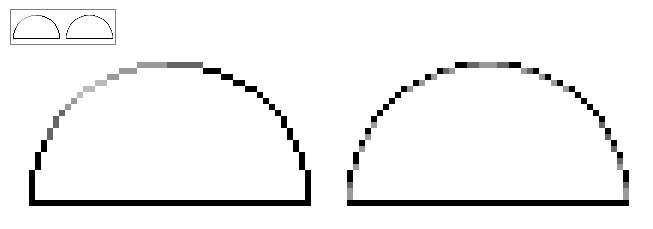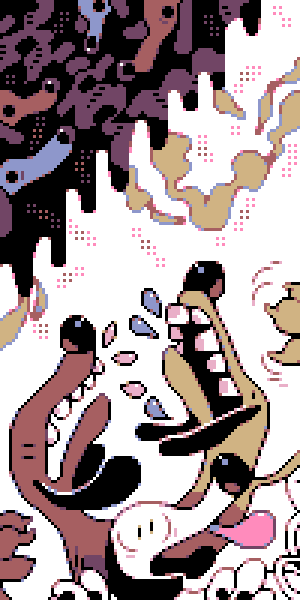Selective Anti-Aliasing is a common technique in pixel art. The idea is to color some parts of the outline to a different color so it blends better with the background color.

Example:The left semicircle is colored with directional lighting. The right one uses selective anti-aliasing to work with all background color.

However, consistently applying anti-aliasing is very time consuming and this procedure can be easily automated.

I wrote this simple algorithm:

1. Given a pixel perfect line
2. Calculate the length and direction of each 1-px-wide chunk
3. For each chunk, color some fraction of the length to some other color

Note: The direction is used to keep track of the coloring orientation: i.e. all vertical chunks have \$orig_color top half, \$alt_color bottom half.

``````/// selectively anti-alias a pixel perfect line
/// each segment of length l contains floor(l*k) number of \$alt_color pixels
pub fn selective_antialias(path: &mut Pixels, k: f64, alt_color: Color) {
let mut chunks = vec![];
let mut start_idx = 0;
for (i, (pi,pj)) in path.iter().zip(path.iter().skip(1)).enumerate() {
// if consecutive pixels not connected
if (pi.point.x != pj.point.x) && (pi.point.y != pj.point.y) {
let start_pix = path.0.get_index(start_idx).unwrap();
let dir = start_pix.dir(&pi);
chunks.push((i - start_idx, dir));
start_idx = i + 1;
}
}
let start_pix = path.0.get_index(start_idx).unwrap();
let dir = start_pix.dir(path.iter().last().unwrap());
chunks.push(((path.len() - start_idx - 1), dir));
assert_eq!(chunks.iter().map(|i|i.0 + 1).sum::<usize>(), path.len());
let mut idx = 0;
for (l, dir) in chunks {
for j in 0..=l {
// xor with dir to keep orientation of coloring
// example:
//           o    o
//           o    o
//           x    x
//           x    x
//            ooxx
if (j <= (l as f64 * k) as usize) ^ dir {
let p = path.0.get_index(idx).unwrap().with_color(alt_color);
path.0.replace(p);
} else {
// noop
}
idx += 1;
}
}
}
``````

This algorithm can be modified to support multi-colored lines.# Image source

These images are from @crrackerjack who uses lots of anti-aliasing.

Image source 0

Image source 1